## MTH211 Foundations of Elementary Math 1### Fun with whole numbers.

Notes on Whole Numbers
Fall 2019

#### § 3.2 Addition and Subtraction

##### Math Activity 3.2: Playing with Blocks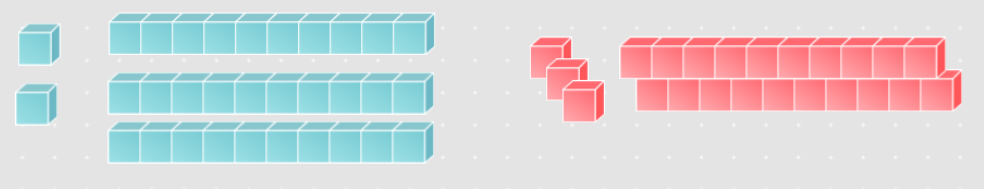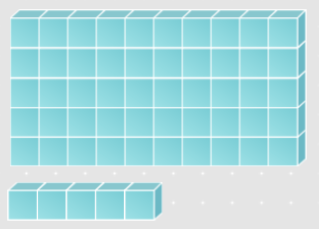##### Math Activity 3.2: Playing with Blocks

Subtraction with base-ten blocks

36 - 23 means, what do you get if you take 23 away from 36?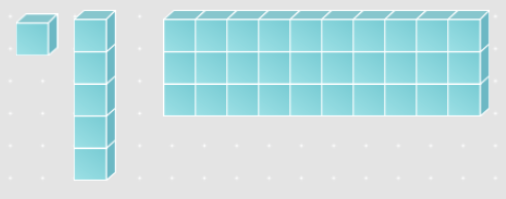Now, take away 23: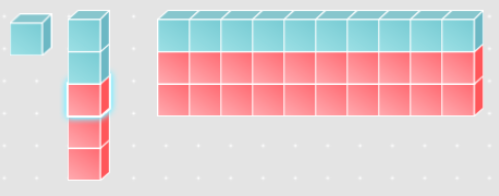That leaves 13!

##### Main topics
• set model
• number line model
• closure
• Commutative
• Associative
• identity
• standard (right to left, regroup)
• Expanded
• Lattice
• Hindu (left to right)
• Inequality
• Subtraction Models
• Take-away
• Compare
• number line
• Subtraction Algorithms
• Standard (right to left, rename)
• Hindu (left to right)
• Complements
• Subtract up
• low stress
• Approximations and Estimations
• Front end
• Compatible numbers

#### § 3.3 Multiplication

Math Activity 3.3: Multiplication as Area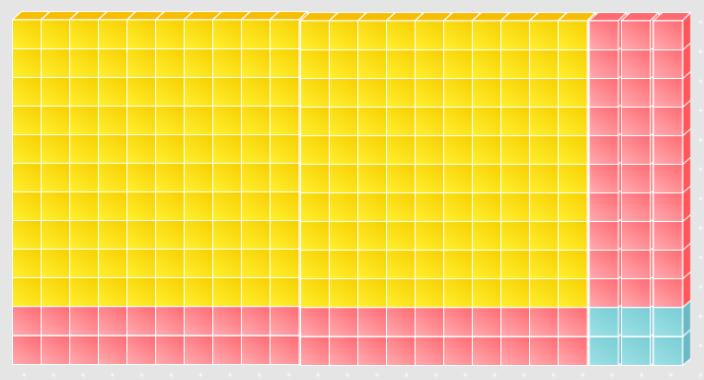##### Main Topics
• Multiplication Models
• Groups of
• Area
• Array
• Cartesian Product
• Factors
• Products
• Partial Products
• Algorithms
• Expanded
• Regrouping (Standard)
• Hindu (left to right)
• Lattice
• Properties
• Closure
• Commutative
• Associative
• Identity (one)
• Distributive property of multiplication over addition
• Estimation

#### § 3.4 Division and Exponents

##### Math Activity 3.4: Division Models

Check out section 3.4 of the book, Division and Exponents. There are 2 models listed:

1. The Set Partition Model (sharing concept)

2. The Repeated Subtraction Model (measurement concept)

For each of these illustrate and explain 42/3. Your illustrations should be similar to those in the book for Example D, 48/4.

##### Main topics
• Definition of division:
If n-:d=q, then q*d=n
6-:2=3 since 3*2=6
• Division Models
• Set Partition
Split 6 into 2 groups. How many are in each group?• Repeated Subtraction
How many groups of 2 are in 6?• Missing Factor (aka what do I multiply by?)
6-:2=square means square xx 2 = 6
• Division Algorithms
• Repeated Subtraction
• Grouped Repeated Subtraction
• Scaffolding
• Long Division
• Short Division
• Division Algorithm Theorem
For any whole numbers n and d, with divisor d!=0, there are whole numbers q and r such That
• n=d*q+r
• 0≤r<d
• Division with zero
• 0//3=0
• 3//0 is undefined
• 0//0 is indeterminate
• Equal quotients
70-:20=7-:2=3 1/2
• Approximate quotients and compatible numbers
31-:16~~30-:15=2
• Rounding and front-end estimation
723-:215~~700-:200=7-:2=3 1/2
• Exponents
• b^0=1 show pattern
• b^1=b show pattern
• a^n*a^m=a^(n+m)
• a^n/a^m=a^(n-m)
• (a^n)^m=a^(n*m)
• (a*b)^n=a^n*b^n
• (a/b)^n=a^n/b^n
• Scientific Notation
• Order of Operations
1. Parentheses (inside)
2. Exponents
3. Multiplication OR Division (left to right)
4. Addition OR Subtraction (left to right)

### Chapter 4: Number Theory

#### § 4.1 Factors and Multiples

Math Activity 4.1: ?

#### § 4.2 Greatest Common Factor and Least Common Multiple

Math Activity 4.2: ?